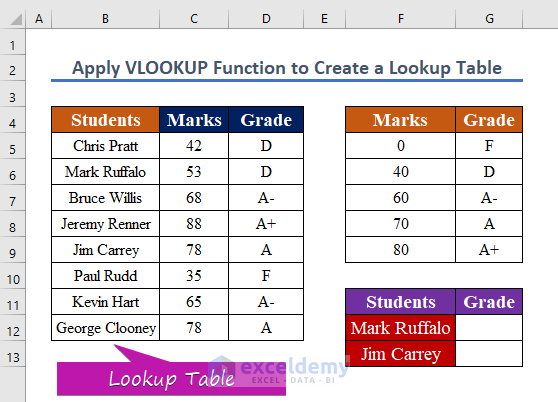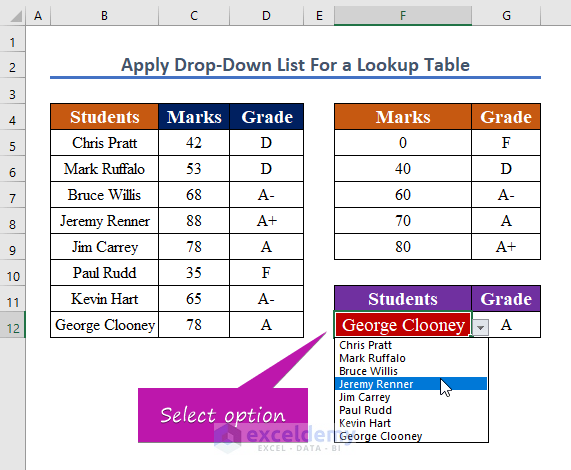Disclosure: This post may contain affiliate links, meaning when you click the links and make a purchase, we receive a commission.

# How to Lookup a Table and Return Values in Excel (3 Simple Ways)

In Excel, lookup tables are named tables that are utilized to find any data when we have a huge volume of data and don’t know where to start finding it. We may name or pick the reference range in the table. In Excel, we may input the table’s name as a reference to look up the value; this sort of table is known as a lookup table. In this tutorial, you will get to know how to use a lookup table in excel applying 3 different functions with examples.

## 3 Simple Ways to Lookup a Table and Return Values in Excel

### 1. Apply the LOOKUP Function to Return Values from a Table in Excel

In Excel, lookup functions are used to search through a single column or row for a certain value. When there are numerous worksheets in a workbook or a big quantity of data on a worksheet, this happens frequently. For instance, we want to know the grade of a particular student and make a data table of their grades. So, we have created a lookup table with grades with the following marks. Now, we will use the lookup table as a reference to look up the grades of the students.Step 1:

`=LOOKUP(C5,\$F\$5:\$G\$9)`

Here,

• C5 is the lookup value.
• \$F\$5:\$G\$9 is the lookup range absolute form.Step 2:

• Then, Press Enter to see the result. Therefore, you will see, the following cell will result as D.Step 3:

• Copy the formula and repeat the steps to look up other values.
• As a result, you will get values of all grades as shown in the below screenshot.

### 2. Combine INDEX and MATCH Functions to Lookup a Table in Excel

The INDEX function in Excel returns the value at a certain position in a range. Whereas, the MATCH function is an Excel function that locates a lookup value’s location in a row, column, or table. The MATCH function is frequently used in combination with the INDEX function to extract a value from a matched place. In the following example, we will find the grade of a certain student and create a lookup table from another lookup table (B5:D12).Step 1:

• To create a lookup table create, enter this formula below in G12.
`=INDEX(D5:D12,MATCH(F12,B5:B12,0))`

Where,

• F12 is the look-up value.
• B5:B12 is the lookup range.
• D5:D12 is the return range.Step 2:

• To see the changes, press Enter. As shown in the below screenshot, you will get the result D.Step 3:

• Now, apply the AutoFill to evaluate other cell values.
• After filling, the final result has been shown in the image below.### 3. Apply Excel VLOOKUP Function to Lookup a Table and Return Values

VLOOKUP is an abbreviation for ‘Vertical Lookup.’ It’s a function that allows Excel to look for a certain value in a column (the so-called ‘table array‘) in order to retrieve a value from another column in the same row. Similar to the previous method, we want to create a lookup table from our lookup table range (B5:D12).

###Step 1:

`=VLOOKUP(F13,\$B\$5:\$D\$12,3, FALSE)`
• F12 is the lookup value.
• \$B\$5:\$D\$ is the absolute lookup range.
• 3 is the column number you want input value.
• We want the EXACT match (FALSE).Step 2:

• To see the changes, press Enter.
• You may obtain values as shown in the below screenshot that, for ‘Mark’ it is showing D.Step 3:

• Copy the formula to other cells, and repeat the process.Note:

In addition to the previous method, you can make a drop-down list to look up a value. To do so, simply follow these steps.

Steps:

• To open a dialog box, press Alt+A+V+V.
• Select List from the options.
• Selects source range \$B\$5:\$B\$12Consequently, you will get a selection box and can select any option you want to lookup.

##Read More: How to Use VLOOKUP Table Array Based on Cell Value in Excel

## Conclusion

To conclude, I hope this article has provided detailed guidance about how to Use the Lookup Table in Excel. You should learn all of these processes and apply them to your dataset. Examine the practice booklet and put your newfound abilities to the test. Because of your invaluable support, we’re inspired to continue creating lessons like this.

Stay with us and continue to learn.

## Related Articles#### Bhubon Costa

Myself, Bhubon Costa, an engineer, is currently employed with Exceldemy as an Excel & VBA Content Developer. I appreciate solving analytical difficulties, finding practical answers, and, for the most part, I enjoy taking on new tasks. I write articles on Exceldemy about various ways to get out of Microsoft Excel's stuck conditions. My passion is to leave my mark on the world through my work and to have an impact on the community who benefit from it.

We will be happy to hear your thoughts